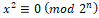# Vol. 3, Issue 2, Part A (2021)

## Formulation of solutions of a special standard quadratic congruence modulo an even prime integer raised to the power n

Author(s):

B M Roy, A A Qureshi

Abstract:
In this paper, authors have formulated solutions of special type of a standard quadratic congruence x2≡0 mod 2nof composite modulus. This congruence was not formulated by the earlier mathematicians, so the present authors studied it rigorously and attempted to formulate the solutions of this congruence. The authors have presented the formula for the solutions of the said quadratic congruence here and obtained nonzero solutions.